# The best programs for solving math problems for Android and iPhone

From students to students, they all struggle with problems to solve different math problems. But with the best programs for solving math problems that are available for Android and iPhone phones, from now on you can solve all problems easily.Maybe solving math questions is one of the most difficult tasks for you and you will do anything to avoid it. But there are different programs and software that you can get help from on your phone and solve your various math problems through them.

Due to the ever-increasing emergence of technology at all levels, applications for solving mathematical problems have been produced for Android smartphones and tablets equipped with the iOS operating system. The best math problem solving programs are able to solve complex math problems easily.

The best note-taking apps for Android and iPhone

The best slow motion app for Android and iOS

## The best programs for solving math problems

It doesn’t matter if your phone’s operating system is Android or iPhone Android math problem solving program and iPhone are both available and you can download the program you want and use it.

Using these programs to solve various problems is very practical and helpful, and from students to students at higher levels, they can also use it.

Below are some examples of math problem solving programs for iPhone and Android. These programs can be used to help solve any problem in mathematics, from the simplest to the most difficult.

You can easily learn algebra, geometry, graphs and matrices and differential with the help of these free programs. Be with us by introducing the best programs for solving math problems.

### Geometry solver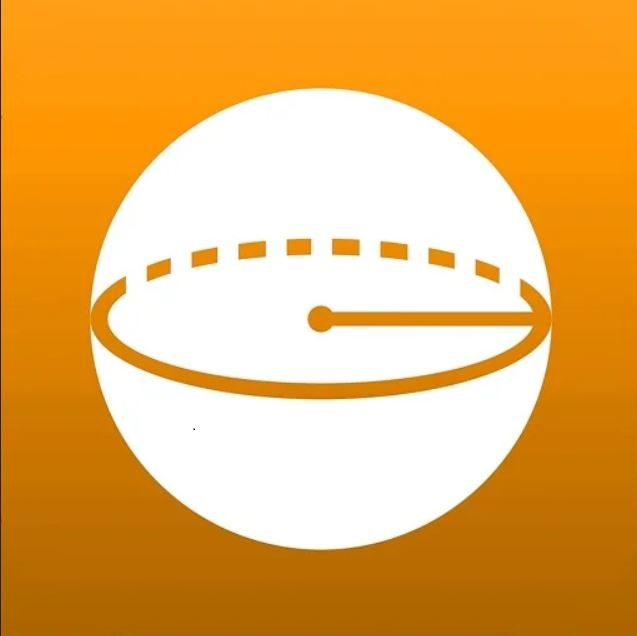this iPhone math problem solving program It can help you very well. This application uses 2D and 3D images that you can use to draw any shape you want and solve your math problems.

Using Geometry solver is not complicated at all and we see a smooth and user-friendly interface that will not cause problems for people, even young students.

### PocketCAS pro 3.6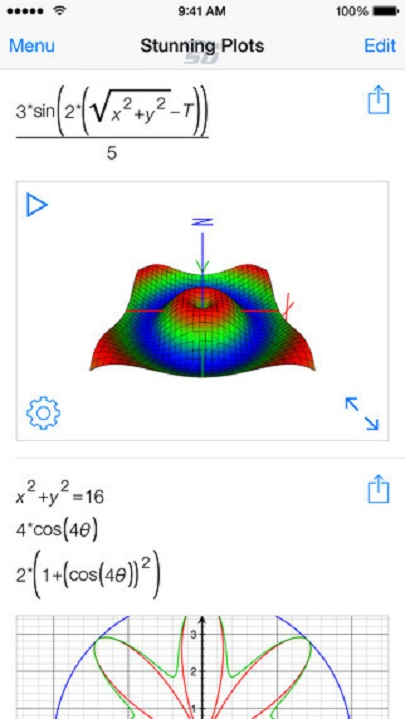PocketCAS pro 3.6 is a software for solving mathematical problems for iPhone, which is very practical. With PocketCAS pro software, you can easily solve math problems from elementary school to college differential and integral.

This software is an essential tool for students, teachers and engineers, which can solve many complex problems while being simple, and is considered a full-fledged calculator.

### Math Scanner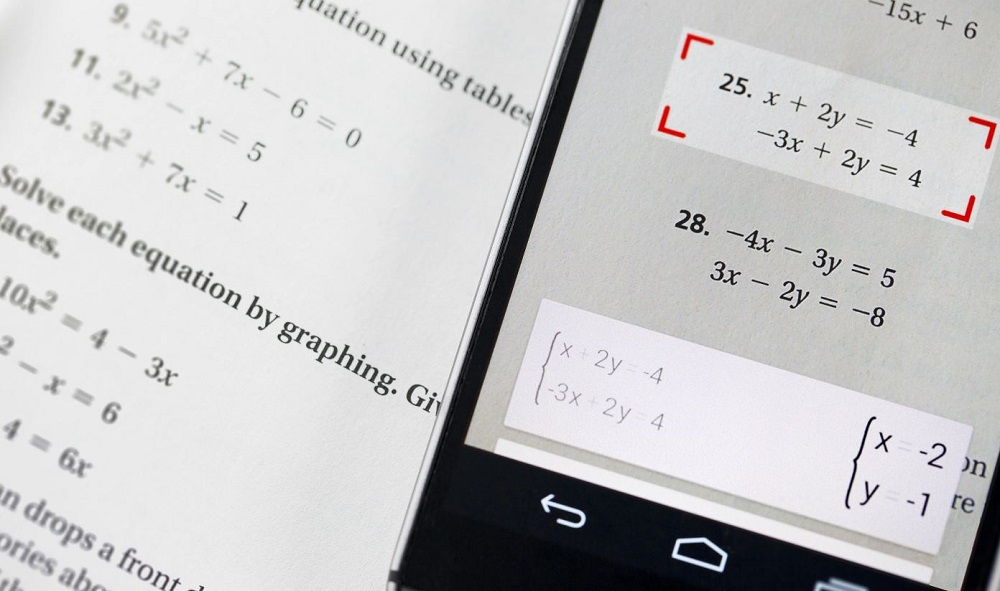If you have problems solving math problems and you want to choose a program to solve math problems, you can download and install the Math Scanner program. Working with Math Scanner is very simple.

There are three ways to enter questions in the Math Scanner program. You can type your math question, you can take a photo with the app’s camera or upload a photo from your gallery. You can solve various problems such as algebra, trigonometry, differential calculus, statistical problems and chemistry using the Math Scanner program.

The best financial management applications

The best Android antivirus 2022

### Chegg Math Solver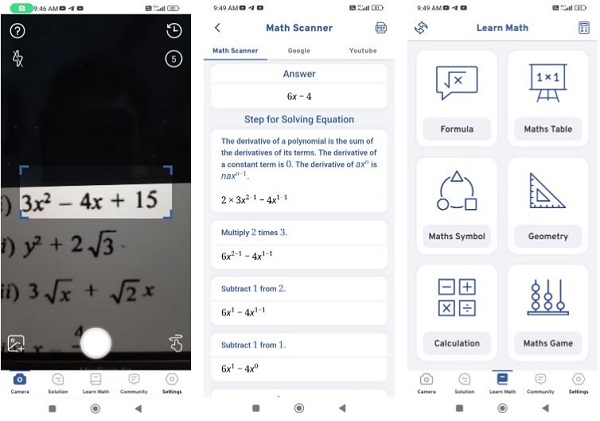Chegg Math Solver is one of the best math problem solving apps that you can use on your Android smartphone. To solve the mathematical equation, users must scan the relevant formula through the device’s camera and take a picture of the question.

When finished, Chegg Math Solver will automatically solve the math equation. The app also shows a step-by-step solution on how to solve the equation.

### CameraMathCameraMath is a photo-based math problem solving program. Take a photo with the device’s camera or select the desired area from the saved photos and receive the answer. The app’s artificial intelligence extracts key information and solves the math problem simply and instantly by providing a solution.

### Maple Calculator: Math Helper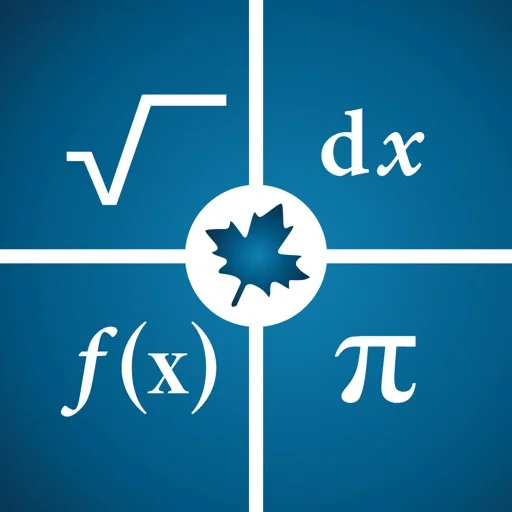Maple Calculator is a free and powerful math problem solving app for Android and iPhone that provides you with 2D and 3D graphs and even step-by-step solutions.

This program makes it very easy to solve math problems, algebra, differential, integral and differential equations, and you can scan the problems using your camera and get the answers to your homework with one click.

The best WiFi hacking program; How to hack Wi-Fi?

The best game hacking program; Enjoy the game without limits

### MyScript Calculator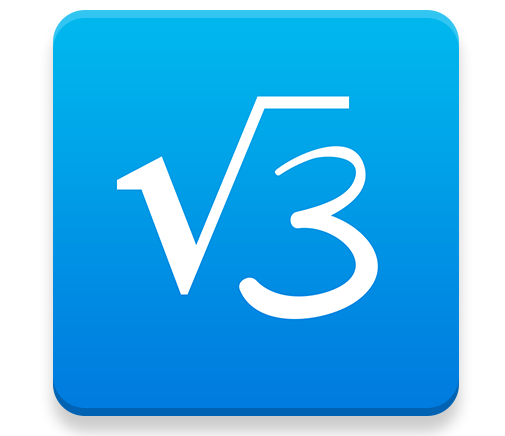Get instant solutions to math problems with the MyScript application. This app for solving math problems on the phone allows the user to enter the math problem into the app and get better solutions for their question. The use of this program is mostly suggested to students, because its use is not particularly complicated.

### Desmos Graphing CalculatorDesmos Graphing Calculator app automatically solves equations for users. This calculator can draw various equations, parabola lines, etc. Another feature of this software is receiving data and drawing graphs. Using Desmos Graphing Calculator, graphs, trigonometric, differential and integral equations and even statistics and probability can be solved.

### GauthmathGauthmath is a free mobile math problem solving app that reads and solves math problems instantly using your smartphone’s camera. Gauthmath uses smart technology to read math problems and provide step-by-step solutions.

### Microsoft Math Solver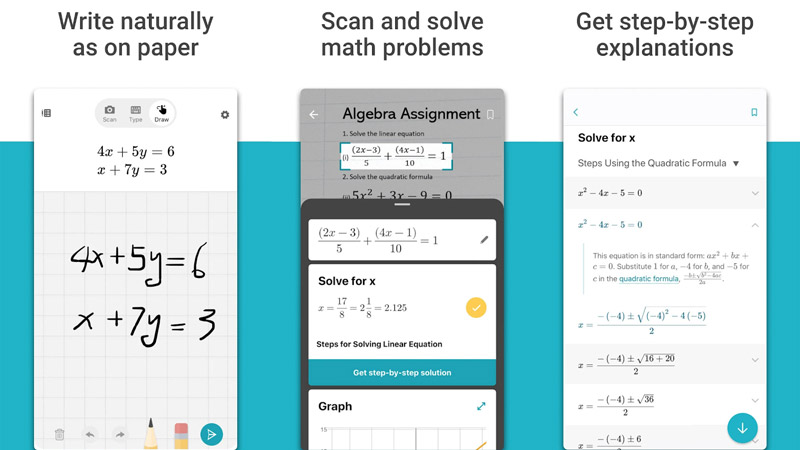The Microsoft Math Solver program was produced by the large American company Microsoft and solves math problems with the help of artificial intelligence. In this program, you can take pictures of math problems with the help of the camera and see how to solve them.

In the program itself, there is a note environment where you can manually write the problems using a pen or finger touch so that the program will give you the answer after analyzing the problem.

### Graphing Calculator + Math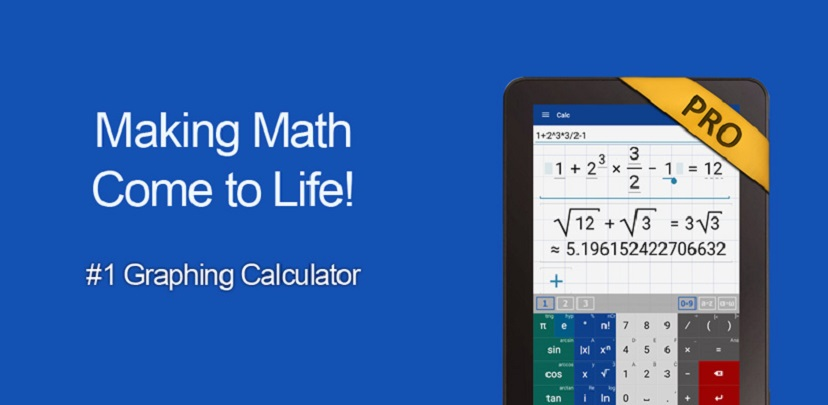This program is a specialized calculator for solving math problems. In the Graphing Calculator + Math program, various calculators are provided to the user to solve all kinds of math problems. With these calculators, it will be very easy to write the problem and solve it.

Also, the answers to math problems are displayed in the form of graphic diagrams to make them easier to understand. Graphing Calculator + Math program has been downloaded more than 5 million times from Google Play and has many fans.

### Photomath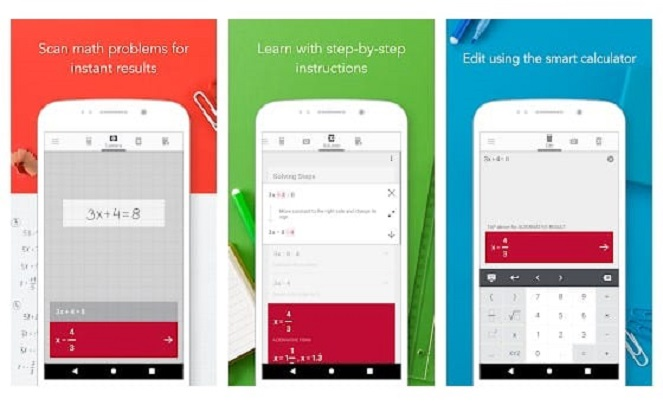This program is one of the best programs for solving math problems. The great feature of Photomatch software is that you can directly take pictures of math problems using your phone’s camera so that the program can solve it by itself. It also tells you step by step how to answer the question. This program is the best program for solving math problems for iPhone and Android.

### WolframAlpha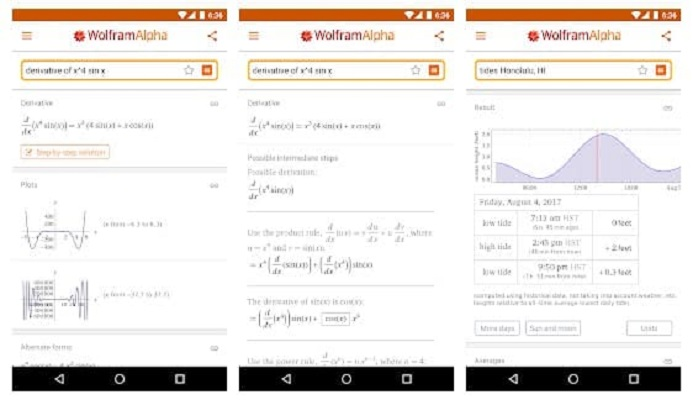WolframAlpha can solve differential and integral equations and calculus. Students from all over the world solve their homework with this app. The range of disciplines that can raise their math questions in this program is large; From pure mathematics and statistics to physics and chemistry, they are all supported by this program with their sub-disciplines.

The best Iranian YouTubers and streamers

### Mathway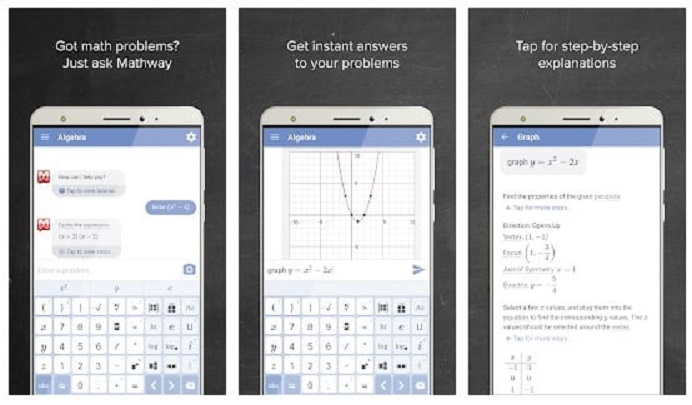The good thing about Mathway app is that it has millions of users worldwide, which means you are connected to more teachers around the world. This software is the best program for solving mathematical problems for Android and it has the possibility of converting the picture of the question into a solved equation. The step-by-step guide to solving the problem is one of the options of this app.

### MalMath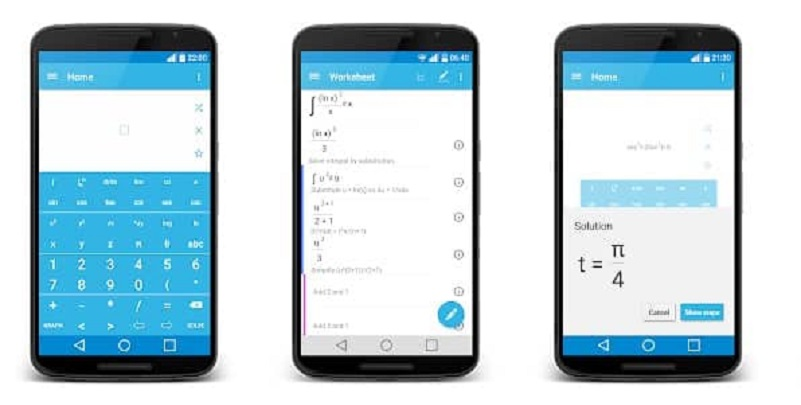MalMath is good for those looking for a simple program to solve their math problems. The great possibility of this app is the ability to solve integral, algebra, derivative, etc. questions. This program also provides you with step-by-step problem solving training and you will enjoy working with it.

### Cymath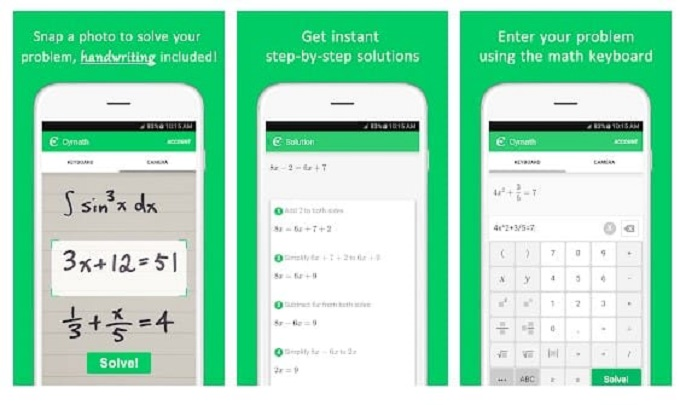Cymath app is the best math problem solving app for Android. Thanks to the advanced engine that this program has, you can solve complex problems. Equations of the first degree, second degree, etc. can be solved with Cymath. With this program, you can both upload the picture of the question and manually type your question to the program with the math keyboard.

How to see WhatsApp messages without the blue tick

The best WhatsApp alternative in Iran; What to install a program similar to WhatsApp?

### Calculator Pro

Take pictures of your math problems, give them to Calculator Pro and get answers. You can also give multi-line problems to this app and ask it for answers. Besides, a simple calculator is also included in the program. Another interesting thing is that the program keeps all the history of your previous calculations so that you can access them later.

### Socratic

The great thing about Socratic software is that it has a lot of step-by-step guides with detailed explanations so that you can solve even the most difficult math problems. The app has more than 5 million active installs, and according to the creators, more than 100 million math questions have been answered with Socratic.

### iMathematics

iMathematics software acts more like a private math teacher and is one of the best programs for solving math problems. Students at different educational levels can enter their questions in the program.

Mathematical formulas and definitions are effectively placed in this program and can be used by everyone. There are many tools and calculators in this program to help you in the problem solving process.

### Math Calculator

This app is both a normal calculator, an engineering calculator and a math problem solver. Solving the problem is done by the usual method of scanning from the picture of the question. This app also helps you solve exercises.

### Qanda

In Qanda, as expected, enter the image of the math problem with the phone’s camera and get the answer. The positive point of this app is the presence of a large number of teachers from the best schools around the world who help you solve math problems with their guidance.

### last word

In this article, we reviewed and introduced the best program for solving math problems for Android and iPhone; Professional and suitable programs that you can use if you have problems solving your math problems. In fact, sometimes solving math problems requires more than a calculator app, and that’s where these apps come in handy.

### What is the good program for solving math problems on Android?

Solving math problems in different fields has become very convenient and easy compared to previous years. Various mathematical problems can be easily solved through applications and software that are intended for this purpose. For this purpose, you can download the desired program for your Android or iOS phone and use it to solve various math problems. There are various programs for Android, among which Qanda, Socratic, Cymath, Photomath, etc. can be mentioned.

.

What do you think about the best math problem solving apps? Please share your thoughts with Tekrto and other users in the comment section.### Average Rating

5 Star
0%
4 Star
0%
3 Star
0%
2 Star
0%
1 Star
0%Previous post The Galaxy S23 release date has been determined; Earlier than expected!Next post Laptop with sliding screen; Interesting patents from Samsung and Intel